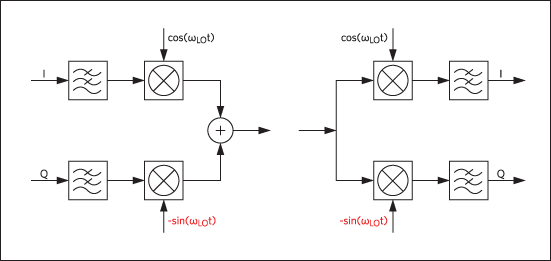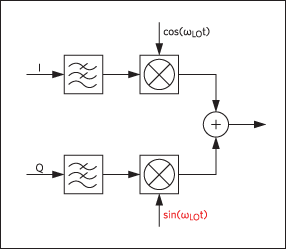# Spectrum Inversion in NCDMA to Ensure Compliance with 3GPP2

### Abstract

Unlike many other cellular standards, NCDMA is required by the 3GPP2 standard to perform spectrum inversion in the physical layer before transmission and after reception. With today’s vast selection of radio frequency (RF) transceivers and baseband processors, it is easy to imagine how one could come across a set of RF transceiver and baseband processor that have mismatched spectrum in the transmit and receive paths. This simple oversight will result in noncompliance to the 3GPP2 standard and failure to demodulate. However, there are a few simple techniques that can help determine if spectrum inversion has been performed on a signal.

A similar version of this article appears on microwaves&rf, October 11, 2013.

### Introduction

This tutorial will help design engineers manage spectrum inversion to comply with the 3GPP2 standard. It is important for system integrators to remember that the 3GPP2 standard requires NCDMA, unlike many other cellular standards, to perform spectrum inversion in the physical layer (generally within the radio frequency (RF) IC) prior to transmission and after reception. With today’s vast selection of RF transceivers and baseband processors, it is easy to imagine how one could find a set of a RF transceiver and baseband processor that has mismatched spectrum in the transmit and receive paths. This simple oversight will result in noncompliance with the 3GPP2 standard and failure to demodulate. However, there are a few simple techniques that can help determine if spectrum inversion has been performed on a signal. This tutorial presents three techniques that perform spectrum inversion on RF transceivers that do not have built-in spectrum inversion.

### How to Detect Spectrum Inversion

In a transmitter, the easiest way to determine if the spectrum has been inverted is by comparing a single-tone continuous wave (CW) with a nominally positive frequency to the local oscillator (LO) frequency. If the RF CW output frequency is greater than the LO frequency (positive offset), then no spectrum inversion has happened. But if the LO frequency is greater than the RF output frequency, then the spectrum has been inverted.
In the receive path, if a positive offset RF input frequency produces an I output that leads the Q output by 90°, then no spectrum inversion was done by the RF demodulator. Generally, the modulation format of the RF demodulator follows the modulator. To demonstrate these points, let’s examine the uplink and downlink paths of a WCDMA system, as specified in the 3GPP standard TS 25.213 (Figure 1).Figure 1. WCDMA I/Q modulation and demodulation format. Note that the Q channel is multiplied by a negative phase LO, i.e., -sin(ωLOt), as shown in red.

### Transmitter Signals

For simplicity, assume that the transmitter baseband I and Q signals are represented by:
Vm = ejωmt = cos(ωmt) + jsin(ωmt)
(Note that this is a positive frequency, complex tone at baseband.)
The transmitter I and Q LOs are represented by:
LOITX = cos(ωLOt) and LOQTX = -sin(ωLOt)
Now notice the negative polarity of the Q LO:
VTX = cos(ωmt)cos(ωLOt) - sin(ωmt)sin(ωLOt)
VTX = ½cos((ωm - ωLO)t) + ½cos((ωm + ωLO)t) - ½cos((ωm - ωLO)t) + ½cos((ωm + ωLO)t)
VTX = cos((ωm + ωLO)t)
As shown in the equations above, a positive modulation baseband signal, in combination with a negative phase LO frequency, produces an RF output frequency which resides above the LO frequency. The result is no spectrum inversion.

On the receiving end, assume that the same transmitted RF signal is received and demodulated with the same LO format as the transmitter:
VRX = cos(ωRXt), with ωRX = ωm + ωLO
VLO = e-jωLOt = cos(ωLOt) - jsin(ωLOt)
VBB = cos(ωRXt)cos(ωLOt) - jcos(ωRXt)sin(ωLOt)
After multiplying out the terms and applying lowpass filtering to remove the higher frequencies:
VBBI = ½cos((ωRX - ωLO)t) and VBBQ = ½sin((ωRX - ωLO)t)
Substituting ωRX with ωm + ωLO, the I and Q baseband outputs are the same as the I and Q of the TX baseband inputs:
Im = cos(ωmt) and Qm = sin(ωmt)

### Perform Spectrum Inversion

Now that we have a clear understanding of how to detect spectrum inversion, we turn our attention to the available methods of achieving it on purpose. As mentioned earlier, the 3GPP2 standard requires that the signal spectrum of NCDMA be inverted prior to transmission and after reception. Following are three simple methods that can be used for spectrum inversion.

### Method 1

Spectrum inversion by adopting a positive phase LO. This is the modulation format recommended by the 3GPP2 standard (Figure 2).Figure 2. NCDMA I/Q modulation format. Note how the Q channel is multiplied by LO with positive phase, i.e., sin(ωmt), as highlighted in red.
Im = cos(ωmt) and Qm = sin(ωmt)
LOITX = cos(ωLOt) and LOQTX = sin(ωLOt)
VTX = cos(ωmt)cos(ωLOt) + sin(ωmt)sin(ωLOt)
VTX = ½cos((ωm - ωLO)t) + ½cos((ωm + ωLO)t) + ½cos((ωm - ωLO)t) - ½cos((ωm + ωLO)t)
VTX = cos((ωm - ωLO)t)
As shown here, the RF output frequency is lower than the LO frequency. Therefore, the spectrum has been inverted.

### Method 2

Spectrum inversion by inverting the polarity of the Q baseband signal.
Im = cos(ωmt) and Qm = -sin(ωmt)
LOITX = cos(ωLOt) and LOQTX = -sin(ωLOt)
VTX = cos(ωmt)cos(ωLOt) + sin(ωmt)sin(ωLOt)
VTX = cos((ωm - ωLO)t)

### Method 3

Spectrum inversion by swapping the I and Q transmit baseband signals.
Im = sin(ωmt) and Qm = cos(ωmt)
LOITX = cos(ωLOt) and LOQTX = -sin(ωLOt)
VTX = sin(ωmt)cos(ωLOt) - cos(ωmt)sin(ωLOt)
VTX = ½sin((ωm + ωLO)t) + ½sin((ωm - ωLO)t) - ½sin((ωm + ωLO)t) + ½sin((ωm - ωLO)t)
VTX = sin((ωm - ωLO)t)

### Conclusion

Method 1 is the method for performing spectrum inversion recommended by the 3GPP2 standard. However, as shown above, other methods can achieve the same result and still be spec compliant. In all cases for parts derived from the MAX2553 WCDMA transceivers, the baseband input and output pins are labeled so that no spectrum inversion occurs in the transceiver, neither in the transmitter path nor in the receiver path. For compliance with the 3GPP2 standard, one should be aware of this and apply one of the above methods for inverting the transmit spectrum. For the receive path, a corresponding inversion is also required in most cases, unless the baseband is already programmed to accept inverted spectrum signals.